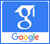# All Articles in May, 2022## Associations of arterial stiffness with left ventricular mass index and carotid intima-media thickness in the hypertensives

Published on: 5th May, 2022

Background: Arterial stiffness has been considered an independent predictor of cardiovascular disease in addition to the traditionally known cardiovascular risk factors. Objectives: This study aimed to investigate the associations between arterial stiffness with left ventricular mass index and carotid intima-media thickness in the hypertensives. Methods: A descriptive cross-sectional study compared a control group in 210 study subjects (105 hypertensives and 105 normotensives). Measuring left ventricular mass index by echocardiography and carotid intima-media thickness by carotid doppler ultrasonography. Pulse wave velocity was measured using the Agedio B900 device and the Agedio K520 application. The manual method was measured by the ankle-brachial index.Results: There was a statistically significant positive correlation between pulse wave velocity and age (r = 0.922, p < 0.001). The ankle-brachial index had a statistically significant positive correlation at a weak level with left ventricular mass index and carotid intima-media thickness, in which the coefficient r was equal to 0.219 (p < 0.05) and 0.250 (p < 0,001), respectively. Pulse wave velocity also had a statistically significant positive correlation at a weak level with left ventricular mass index and carotid intima-media thickness, in which the coefficient r was equal to 0.188 (p < 0.05) and 0.289 (p < 0,001), respectively. Pulse wave velocity had a multivariable linear correlation with gender, pulse, mean blood pressure, and ankle-brachial index with statistical significance; and they were written in the form of the following equation: Pulse wave velocity (R2: 41.3%) = 0.641*(Gender) – 0.027*(Pulse) + 0.043*(Mean blood pressure) + 8.378*(Ankle-brachial index) – 3.254.Conclusion: Arterial stiffness was statistically correlated with left ventricular mass index and carotid intima-media thickness in the hypertensives. Through the above research results, we suggest that the hypertensives should be combined with the evaluation of hemodynamic parameters and arterial stiffness for contributing to the diagnosis and detection of cardiovascular complications, thereby improving the quality of monitoring and treatment in hypertensive patients.